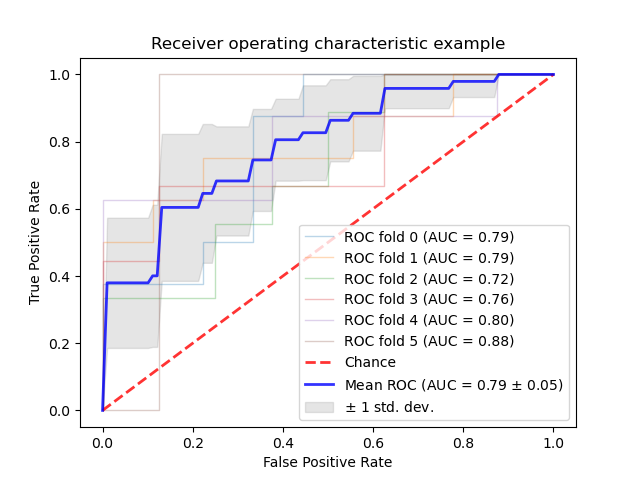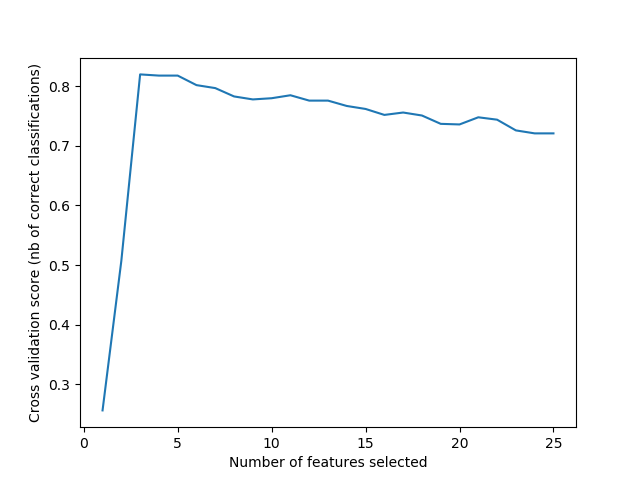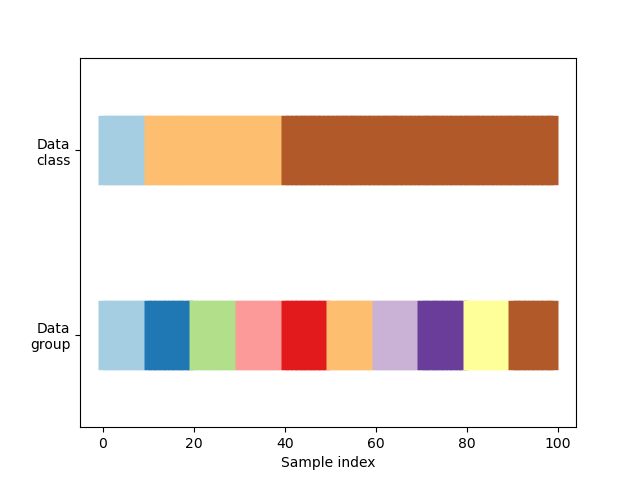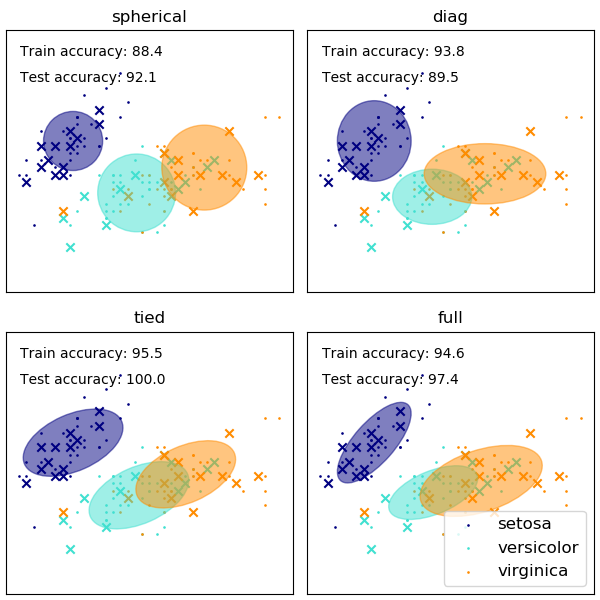# sklearn.model_selection.StratifiedKFold¶

class sklearn.model_selection.StratifiedKFold(n_splits=5, *, shuffle=False, random_state=None)

[源码]

n_splits int, default=5

shuffle bool, default=False

random_state int or RandomState instance, default=None
shuffle为True时，random_state会影响索引的顺序，从而控制每个类别的每一折的随机性。否则，保留random_stateNone。为多个函数调用传递可重复输出的int值。请参阅词汇表

• 生成测试集，使它们全部包含相同的类分布，或尽可能接近。
• 类标签不变：重新将y = ["Happy", "Sad"]标记为y = [1, 0]，不应更改生成的索引。
• shuffle=False时，保留数据集排序中的顺序依赖关系 ：在一些测试集中，类别k中的所有样本在y中是连续的，或在y中被除k以外的类别的样本所分隔。
• 生成最小和最大相差最多一个样本的测试集。

>>> import numpy as np>>> from sklearn.model_selection import StratifiedKFold>>> X = np.array([[1, 2], [3, 4], [1, 2], [3, 4]])>>> y = np.array([0, 0, 1, 1])>>> skf = StratifiedKFold(n_splits=2)>>> skf.get_n_splits(X, y)2>>> print(skf)StratifiedKFold(n_splits=2, random_state=None, shuffle=False)>>> for train_index, test_index in skf.split(X, y):...     print("TRAIN:", train_index, "TEST:", test_index)...     X_train, X_test = X[train_index], X[test_index]...     y_train, y_test = y[train_index], y[test_index]TRAIN: [1 3] TEST: [0 2]TRAIN: [0 2] TEST: [1 3]

get_n_splits(self[, X, y, groups]) 返回交叉验证器中的切分迭代次数
split(self, X, y[, groups]) 生成索引以将数据分为训练集和测试集。
__init__（self，n_splits = 5，*，shuffle = False，random_state = None ）

[源码]

get_n_splits（self，X = None，y = None，groups = None ）

[源码]

X object

y object

groups object

n_splits int

split（self，X，y，groups = None ）

[源码]

X array-like of shape (n_samples, n_features)

y array-like of shape (n_samples,)

groups object

train ndarray

test ndarray

## sklearn.model_selection.StratifiedKFold使用示例¶